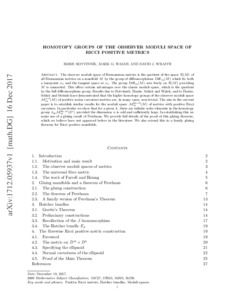# Homotopy groups of the observer moduli space of Ricci positive metrics

Botvinnik, Boris and Walsh, Mark G. and Wraith, David J. (2019) Homotopy groups of the observer moduli space of Ricci positive metrics. Geometry & Topology, 23. pp. 3003-3040. ISSN 1465-3060Previewmore...Add this article to your Mendeley library

## Abstract

The observer moduli space of Riemannian metrics is the quotient of the space R(M) of all Riemannian metrics on a manifold M by the group of diffeomorphisms Diffx0(M) which fix both a basepoint x0 and the tangent space at x0. The group Diffx0(M) acts freely on R(M) provided that M is connected. This offers certain advantages over the classic moduli space, which is the quotient by the full diffeomorphism group. Results due to Botvinnik, Hanke, Schick and Walsh, and Hanke, Schick and Steimle have demonstrated that the higher homotopy groups of the observer moduli space Ms>0x0(M) of positive scalar curvature metrics are, in many cases, nontrivial. The aim in the current paper is to establish similar results for the moduli space MRic>0x0(M) of metrics with positive Ricci curvature. In particular we show that for a given k, there are infinite-order elements in the homotopy group π4kMRic>0x0(Sn) provided the dimension n is odd and sufficiently large. In establishing this we make use of a gluing result of Perelman. We provide full details of the proof of this gluing theorem, which we believe have not appeared before in the literature. We also extend this to a family gluing theorem for Ricci positive manifolds.

Item Type: Article positive Ricci curvature; moduli space; Riemannian metrics; Perelman gluing construction; Hatcher bundles; Faculty of Science and Engineering > Mathematics and Statistics 12071 https://doi.org/10.2140/gt.2019.23.3003 Dr. David Wraith 02 Jan 2020 13:19 Geometry & Topology Mathematical Sciences Publishers (MSP) YesItem control page